Function Graphing in 2D and 3D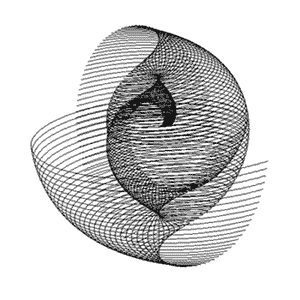3D polar graph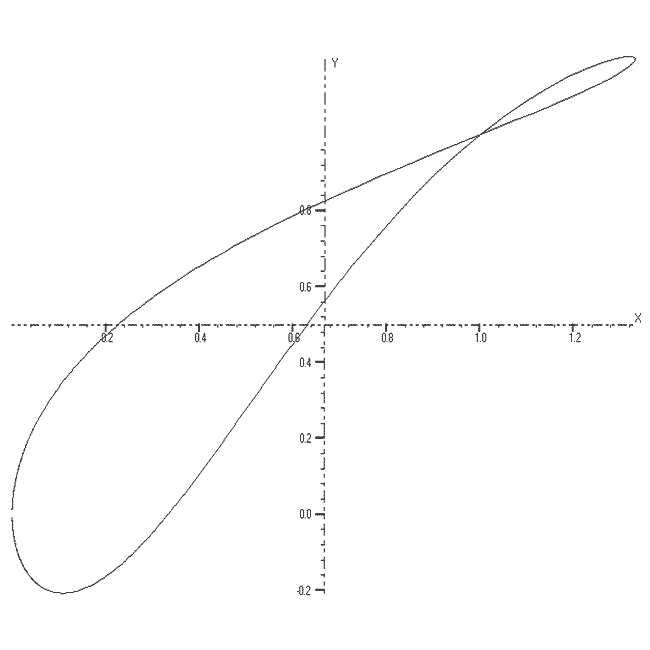2D Parametric function
The GRAPHING package allows you to draw graphs for nine types of function.

These are functions of the form:-

• z = f(x, y),
• x = f1(u,v), y = f2(u,v), z = f3(u,v)
• r = f(a, b),
• x = f1(t), y = f2(t), z = f3(t)
• r = f1(t), a = f2(t), z = f3(t)
• z = f(r, a),
• y = f(x),
• r = f(a) and
• x = f1(t), y = f2(t)

The functions can be plotted without or with axes which can be scaled and the resolution of the graph can be changed to facilitate speed of plotting.

Up to 10 different functions of the same type can be overlaid with each being drawn in a different colour.

The 3D functions can be displayed and printed in one of two stereo forms: stereo-pairs or red/blue anaglyphs.

Functions can be stored and any number of different storage files created. There is also an extensive "Help" file included.

The package now allows 3D surface plotting with some forms of graph, courtesy of the Surface Viewer control by Bright Ideas Software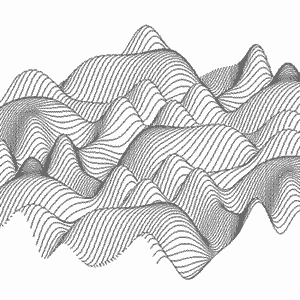z = f(x,y) with hidden lines removed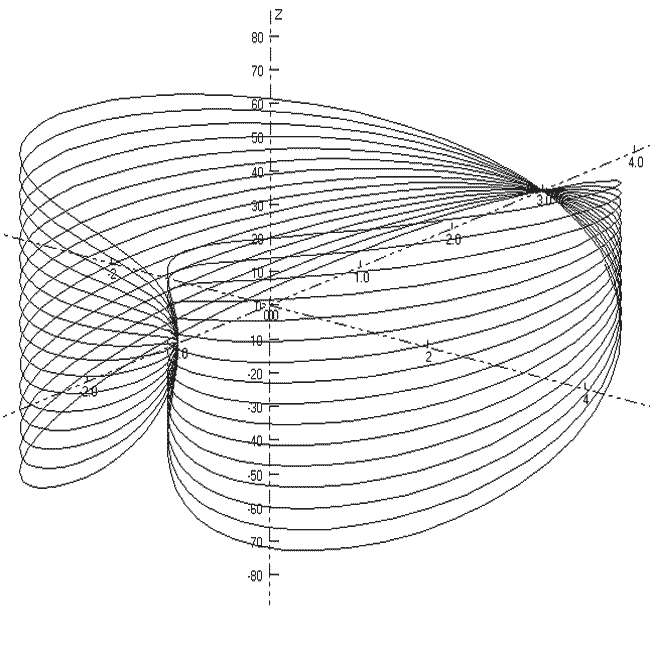3D Parametric function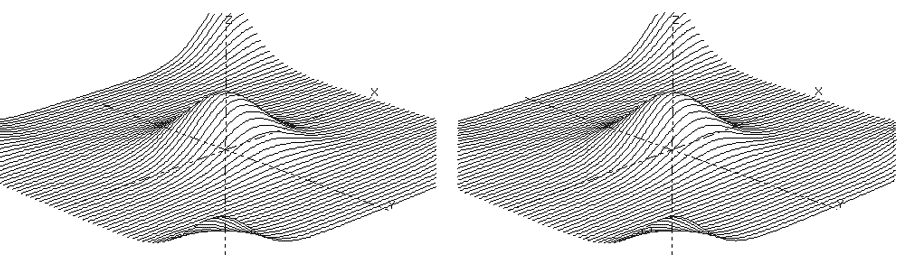Stereo-pair

### or download the GRAPHING package, (3.38M).

Other graphing sites:-

Open GL 3D graphing
Pacific Tech
3D theory
Study Works, 3D graphing
Curvus Pro - the reference for 2D curves and 3D graphs
Bright Ideas software, 3D graphs and calculus software
Cool graphs